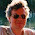Montag, 4. Januar 2010

Use PowerShell to get openfiles with handle.exe

You find handle.exe at http://technet.microsoft.com/en-us/sysinternals/bb896655.aspx.

You can use the following script to store a snapshot of the open files in a grid. You have to adapt the path to handle.exe.

C:\Usr\sysint\handle.exe | foreach{
if (\$_ -match '^(?<program>\S*)\s*pid: (?<pid>\d*)\s*(?<user>.*)\$') {
\$matches | %{
\$id = \$_.pid
\$program = \$_.program
\$user = \$_.user
}
}
if (\$_ -match '^\s*(?<handle>[\da-z]*): File \((?<attr>...)\)\s*(?<file>(\\\\)|([a-z]:).*)') {
#\$_
\$matches | select @{n="Pid";e={\$id}}, @{n="Program";e={\$program}}, @{n="User";e={\$user}}, @{n="Handle";e={\$_.handle}}, @{n="attr";e={\$_.attr}}, @{n="Path";e={\$_.file}}
}

} | out-Gridview

I would like to fetch just the files in the current disk queue.

2010-01-16 Edited. I had a problem with html escape characters. Thanks for the comment.

Kommentare:

1.Hallo,
etwas ist falsch.
Error -> BadOperatorArgument for -match

-----
if (\$_ -match "^(?\S*)\s*pid: (?\d*)\s*(?.*)\$")
...
if (\$_ -match '^\s*(?[\da-z]*): File \((?...)\)\s*(?:(\\\\)|([a-z]:).*)')
...

Danke für diese script.

2.You are right. The problem is caused by Copy_ColoredAsHtml, which didn't escape the '<' and '>'. Therfore the groupnames
und and are not shown behind the question marks.
I'm trying to post a correcet version.

3.Sorry they are not show in the above Komment too.

4.Next try the missing groups are:

<program>
<pid>
<user>
<handle>
<attr>
<file>